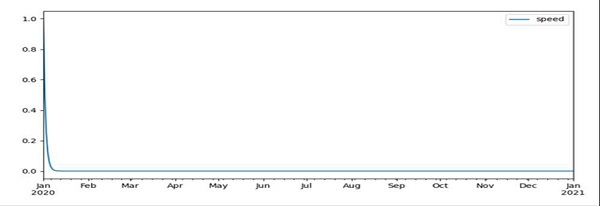# Pandas timeseries plot setting X-axis major and minor ticks and labels

Using Pandas, we can create a dataframe with time and speed, and thereafter, we can use the data frame to get the desired plot.

## Steps

• Construct a new Generator with the default BitGenerator (PCG64).

• Using Pandas, get a fixed frequency DatetimeIndex. From '2020-01-01' to '2021-01-01'.

• Draw samples from a log-normal distribution.

• Make a data frame with above data.

• Using panda dataframe create plot, with figsize = (10, 5).

• To show the figure, use the plt.show() method.

## Example

import numpy as np
import pandas as pd
from matplotlib import pyplot as plt

rng = np.random.default_rng(seed=1)
date_day = pd.date_range(start='2020-01-01', end='2021-01-01', freq='D')
traffic = rng.lognormal(sigma=2, size=date_day.size)
df_day = pd.DataFrame(dict(speed=[pow(2, -i) for i in range(len(date_day))]),
index=date_day)

df_day.plot(figsize=(10, 5))

plt.show()

## Output